# Make a loop at the K-Th position in the Linked list

## Introduction to the problem statement

Given a linked list and an integer K. Make a loop at the K-th position, i.e. attach the last node of the linked list to the K-th node from the starting of the list.

Examples:

Input1: 4 6 1 3 9 , K=2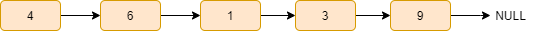Output1: 4 6 1 3 9 6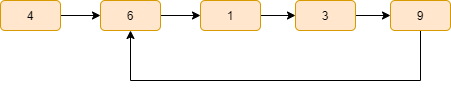Input2: 1 5 6 7 8, K=3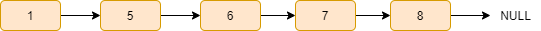Output2: 1 5 6 7 8 6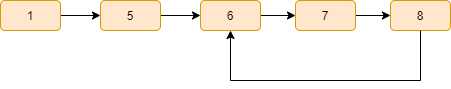## Approach

1. First, reach the K-th node from the starting node of the given linked list.
2. Save the K-th node’s address in a pointer variable.
3. Reach the ending node of the linked list.
4. Attach the ending node with the K-th node of the linked list.

### Steps

1. Declare a curr pointer pointing initially to the head of the linked list.
2. Run a for loop K-1 times and do curr=curr->next.
3. Now, the curr pointer is pointing to the K-th node of the linked list. Declare another pointer kth_pos and point it to the K-th node of the linked list, i.e. kth_pos=curr.
4. Now, Run a while loop till curr->next!=NULL and inside the while loop, perform curr=curr->next.
5. Now, the curr pointer is pointing to the ending node of the linked list.
6. Finally, attach the end node to the K-th node of the linked list, i.e. curr->next=kth_pos.

Let’s understand the above algorithm with an example:

Input: 4 6 1 3 9, K=3

Initially, the curr pointer is pointing to the head of the given linked list.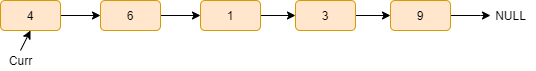The curr pointer reaches the K-th node of the linked list. Now, point this K-th node with another kth_pos pointer.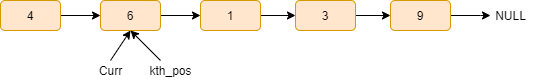Move the curr pointer by one step, curr=curr->next.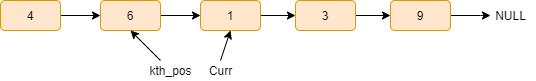Move the curr pointer by one step, curr=curr->next.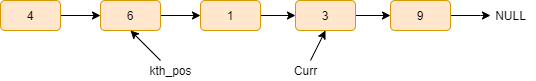Now, here curr->next=NULL, it means curr pointer reaches the end node of the linked list.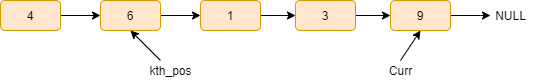Finally, attach the end to the K-th node of the linked list, i.e. curr->next=kth_pos.### Code in C++

``````#include <bits/stdc++.h>
using namespace std;

struct Node {

int data;

Node* next;

Node(int x)
{
data = x;
next = NULL;
}
};

void makeloop( Node* head, int K)
{

for (int i = 1; i < K; i++)
curr = curr->next;

Node* kth_pos = curr;

while (curr->next != NULL)
curr = curr->next;

curr->next = kth_pos;
}

void printList( Node* head, int nodes)
{

for (int i = 0; i < nodes; i++)
{
cout << curr->data << " ";
curr = curr->next;
}

}
{

int count = 0;

while (curr != NULL)
{
count++;
curr = curr->next;
}

return count;
}

int main()
{

int k = 2;

return 0;
}``````

Output

``4 6 1 3 9 6``

### Complexity Analysis

Time complexity- It is O(n), where n is the number of nodes in the given linked list as we are visiting all the nodes.

Space complexity- The space complexity of the above solution is O(1) as we need constant extra space.

Q1. What is a linked list?

Ans: A linked list is a dynamic data structure in which each element (called a node) consists of two components: data and a reference (or pointer) to the next node. A linked list is a collection of nodes, each of which is linked to the next node by a pointer.

Q2. Does the linked list have a loop?

Ans:  A loop in a linked list is a condition that occurs when there is no end to the linked list. When a loop exists in a linked list, the last pointer does not point to the Null as in a singly or doubly linked list or the head of the linked list as in a circular linked list.

Q3. What are the types of linked lists?

## Key Takeaways

So, this article discussed the approach to make a loop at the K-th position in the linked list with a complete explanation and its implementation in C++.

If you are a beginner, interested in coding and want to learn DSA, you can look for our guided path for DSA, which is free!

In case of any comments or suggestions, feel free to post them in the comments section.